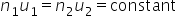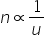# Physical Quantity

Physical Quantity.

A quantity which can be measured and by which various physical phenomenon can be explained and expressed in form of laws of physics is called a physical quantity. For example length, mass, time, force etc.

Measurement is a process in which a physical quantity is compared with its unit.

Measurement is a comparison process.

Measurement is necessary to determine magnitude of a physical quantity, to compare two similar physical quantities and to prove physical laws or equations.

Representation: A physical quantity is represented completely by its magnitude and unit. For example, 100 metre means a length which is hundred times the unit of length 1 kg. Here 100 represents the numerical value of the given quantity and metre represents the unit of quantity under consideration. Thus in expressing a physical quantity we choose a unit and then find that how many times that unit is contained in the given physical quantity, i.e.

Physical quantity  (Q) = Magnitude × Unit  = n × u

n represents the numerical value and u represents the unit. Thus while expressing definite amount of physical quantity.

Note: as the unit(u) changes, the magnitude(n) will also change but product ‘nu’ will remain same.

i.e.               n u  = constant,          orthereforei.e. Larger the unit, smaller will be the magnitude.

Types of Physical Quantity.

(1) Scalar  : These quantities which have only magnitude and do not have any direction e.g. Length, time, work, energy etc.

Magnitude of a physical quantity can be negative. Scalar quantities can be added or subtracted with the help of following ordinary laws of addition or subtraction.

(2) Vector  : These are those quantities which have both magnitude and direction. e.g. displacement, velocity, acceleration, force etc.

Vector physical quantities can be added or subtracted according to vector laws of addition. These laws are different from laws of ordinary addition.

Note :   There are certain physical quantities which behave neither as scalar nor as vector. For example, moment of inertia is not a vector as by changing the sense of rotation its value is not changed. It is also not a scalar as it has different values in different directions (i.e. about different axes). Such physical quantities are called Tensors.

Related Keywords